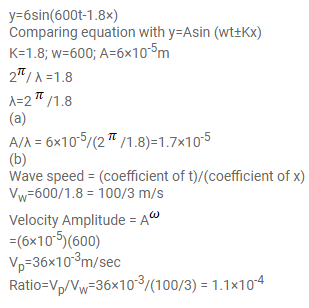# The equation of a travelling sound wave is

Question:

The equation of a travelling sound wave is $y=6.0 \sin (600 t-1.8 x)$ where $y$ is measured in $10^{-5} \mathrm{~m}, t$ in second and $x$ in metre.

(a) Find the ratio of the displacement amplitude of the particles to the wavelength of the wave.

(b) Find the ratio of the velocity amplitude of the particles to the wave speed

Solution: